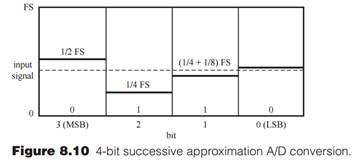Create an Account

Home / Questions / Generate a plot similar to Figure 810 for a 5bit converter if the input signal is 2 25 V a...

Generate a plot similar to Figure 810 for a 5bit converter if the input signal is 2 25 V and the input range is

Generate a plot similar to Figure 8.10 for a 5-bit converter if the input signal is 2.25 V and the input range is -5 V to 5 V. What is the correct digital output?Jul 26 2020 View more View LessSubscribe To Get Solution